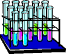Dr. Herrick's CH223 Pressure Quiz 6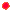2nd Law Thermodynamics Copyright  D. Herrick20 Questions from past exams. Practice for speed.  Aim for 2 minutes per problem.
 1 Thermodynamics says the maximum work by a system occurs
 A) during a spontaneous process at constant pressure. B) at constant temperature. C) at constant volume. D) when there is a catalyst present. E) along a reversible pathway.
 2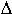So for the reaction2 H2O(g)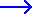O2(g) + 2 H2(g) is expected to be:
 A) positive B) zero C) negative
 3 CalculateGo (kJ) for the reaction at 25C N2O(g)N2(g) + 0.5 O2(g) ,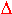Ho =  - 81.6 kJSo = + 75 J/K
 A) -84 B) -104 C) -59 D) -80 E) -1957
 4So for the reactionC2H2(g) + 2.5 O2(g)2 CO2(g) +  H2O(g) is expected to be:
 A) positive B) zero C) negative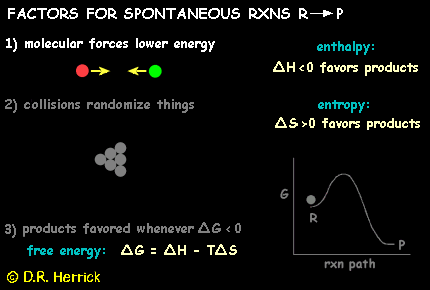5 The total entropy of a system and its surroundings always increases in a spontaneous process.  This is a statement of:
 A) the first law of thermodynamics. B) the law of conservation of matter. C) the second law of thermodynamics. D) the third law of thermodynamics. E) the law of constant composition.
 6 A reaction R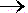P hasHo = - 60 kJ andSo = -100 J/K. The spontaneous reaction favors:
 A) products at all temperatures. B) reactants at all temperatures. C) products at high temperatures, reactants at low temperatures. D) products at low temperatures, reactants at high temperatures.
 7 DetermineGo (kJ) for the reaction at 25C: H2(g) + Cl2(g)2 HCl(g) ,     KP = 2.57 × 1033
 A) 191 B) 95 C) zero D) -95 E) -191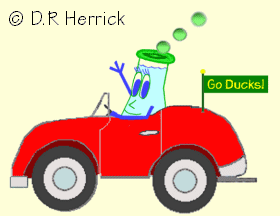8 CalculateGo (kJ) for the reaction at 25C Al(s) + Fe2O3(s)2 Fe(s) + Al2O3(s),Ho = - 484 kJSo = - 39 J/K
 A) -503 B) -496 C) -472 D) -248 E) 5569
 9 DetermineGo (kJ) for the reaction at 25C: AgCl(s)Ag+(aq)  +  Cl-(aq),     K = 1.80 ×10-10
 A) 4.66 B) 55.6 C) 24.1 D) -55.6 E) -24.1
 10 Thermodynamics says the heat absorbed by a chemical reaction at constant T and P is:
 A) zero B) the work done by the system along a reversible pathway C) the enthalpy change for the system D) the entropy change for the system E) the free-energy change for the system
 11 Thermodynamics says a spontaneous chemical reaction at constant T and P always has:
 A) a negative change in the enthalpy of the system. B) a negative change in the entropy of the system. C) a negative change in the internal energy of the system. D) a positive change in the entropy of the system. E) a negative change in the free-energy of the system.12S for the reaction  2 CH3OH(g) + 3 O2(g)2 CO2(g) + 4 H2O(g) is expected to be:
 A) positive B) zero C) negative
 13S for the reaction2 CH3OH(g) + 3 O2(g)2 CO2(g) + 4 H2O(l ) is expected to be:
 A) positive B) zero C) negative
 14 The reactants and products in a chemical reaction at constant T and P are in a state of equilibrium whenever the:
 A) enthalpy change from reactants to products satisfiesH = 0 B) entropy change from reactants to products satisfiesS = 0 C) free-energy change from reactants to products satisfiesG = 0 D) reaction hasH = 0 andS > 0 E) reaction hasH = 0 andS < 0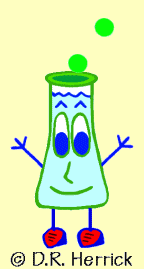15 A reaction RP hasHo = 104 kJ andSo = 88 J/K when reactants and products are in their standard states.  At what temperature willGo = 0 ?
 A)Go cannot be zero for this reaction. B) 909C C) -272C D) 884C E) 25C
 16 According to the reaction data CaCO3(s)Ca 2+ (aq)  +  CO32– (aq) ,Ho = -12.4 kJ ,So = -201 J/K the solubility of calcium carbonate in water between 0C and 100C should be:
 A) weak in cold water; weaker in hot water. B) strong in cold water; strong in hot water. C) weaker in cold water; weak in hot water. D) strong in cold water; weak in hot water. E) weak in cold water; strong in hot water. F) completely insoluble at all temperatures.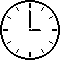Questions 17-20 involve the reaction CaCO3(s)CaO(s)  +  CO2 (g) ,Ho = 178.3 kJ ,So = 159.0 J/K which has a thermodynamic equilibrium constant K = Kp.
 17 The equilibrium pressure (atm) of CO2 at 25C is:
 A) 0.054 D) 2.17 B) 1.1 × 10-23 E) 1.8 × 10-4 C) 9.75 F) 18.2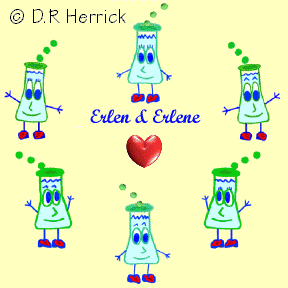18 The equilibrium pressure (atm) of CO2 at 500C is:
 A) 0.054 D) 2.17 B) 1.1 × 10-23 E) 1.8 × 10-4 C) 9.75 F) 18.2
 19 The equilibrium pressure (atm) of CO2 at 1000C is:
 A) 0.054 D) 2.17 B) 1.1 × 10-23 E) 1.8 × 10-4 C) 9.75 F) 18.2
 20 The temperature (C) at which the equilibrium pressure of CO2 is 1.00 atm is:
 A) -21 B) 159 C) 848 D) 1273 E) 467